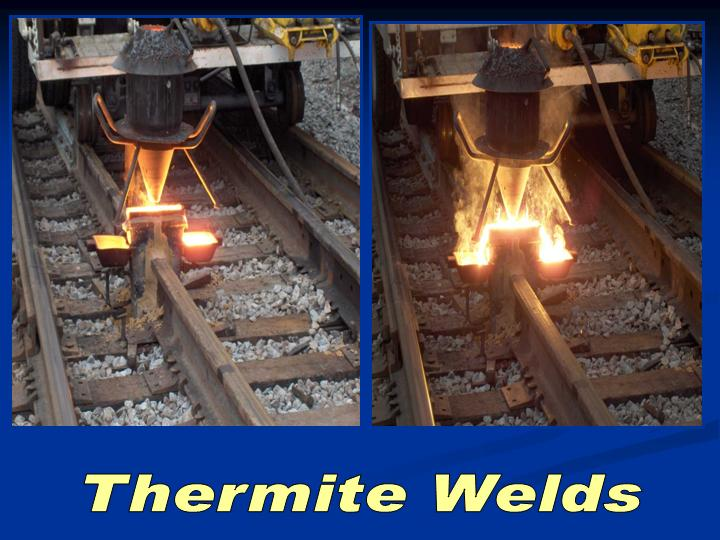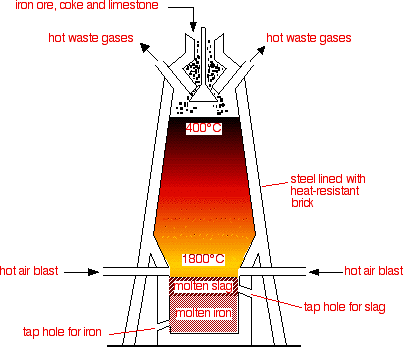# What are the applications of displacement reactions?

Apr 12, 2014

Some applications of displacement reactions are thermite welding, steel making, extraction of metals, and relief from acid indigestion.

#### Explanation:

A single displacement reaction is often of the type

$\text{A + BC → B + AC}$

In this reaction, element $\text{A}$ replaces element $\text{B}$ in the compound $\text{BC}$.

Here are a few examples of the practical applications of displacement reactions.

Thermite Welding

A mixture of $\text{Al}$ and ${\text{Fe"_2"O}}_{3}$ reacts to produce molten iron that is used to join railway rails together.

$\text{2Al" + "Fe"_2"O"_3 → "Al"_2"O"_3 + "2Fe}$

Al replaces $\text{Fe}$ in ${\text{Fe"_2"O}}_{3}$ to form ${\text{Al"_2"O}}_{3}$."CADWELD"^® process

A mixture of $\text{Al}$ and $\text{CuO}$ is used for creating electric joints between $\text{Cu}$ and steel.

${\text{2Al" + "3CuO" → "3Cu" + "Al"_2"O}}_{3}$

$\text{Al}$ replaces $\text{Cu}$ in the $\text{CuO}$.Steel Making

We can extract iron from its ore (${\text{Fe"_2"O}}_{3}$) by heating it with carbon in giant blast furnaces.

${\text{3C" + "2Fe"_2"O"_3 → "4Fe" + "3CO}}_{2}$

$\text{C}$ replaces $\text{Fe}$ in ${\text{Fe"_2"O}}_{3}$ to form ${\text{CO}}_{2}$.Extraction of Metals

Heating a mixture of ${\text{Cr"_2"O}}_{3}$ and finely divided $\text{C}$ produces metallic chromium.

${\text{3C" + "2Cr"_2"O"_3 → "4Cr" + "3CO}}_{2}$

$\text{C}$ replaces $\text{Cr}$ in ${\text{Cr"_2"O}}_{3}$ to form ${\text{CO}}_{2}$ and chromium metal.

Acid Indigestion

We can relieve the distress when the stomach produces too much $\text{HCl}$ by drinking a solution of sodium hydrogen carbonate.

${\text{HCl" + "NaHCO"_3 → "NaCl" + "H"_2"CO}}_{3}$

The $\text{H}$ in the $\text{HCl}$ displaces the $\text{Na}$ in ${\text{NaHCO}}_{3}$ to produce ${\text{H"_2"CO}}_{3}$.